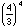# Rationalize Pi

It is possible to approximate pi with a rational number. Let’s play a game where you try to approximate pi to 4 decimal places using an expression of integers (decimals not allowed) with a fraction, exponent, or combination of the two.

The goal will be to get the lowest score possible. Here’s how you keep score: +1 for each digit used, and +0.2 for each ten-thousandth that you are away from the actual value of pi (= 3.1416, to the nearest 4 decimal places.)

For example, 314/100 is equal to 3.14; your score would be +6 for using six digits and +3.2 for being 16 ten-thousandths away from pi, giving a total of +9.2. Another example is; your score would be +3 for using three digits and +37.8 for being 189 ten-thousandths away from pi.

What is the lowest score you can find?